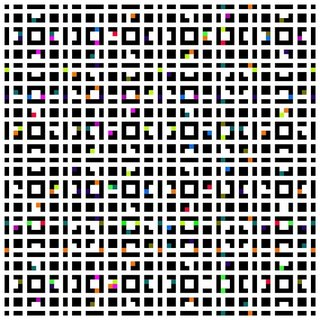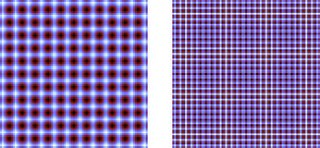Irrational ExuberanceA number (real or complex) can be rational or irrational. A rational number is one that can be written as the ratio of two integers, such as 1/4, -2/11, or (1 + i)/(4 – 5i). When written as a decimal, a rational number either terminates (like 1/4 = 0.25) or repeats (-2/11 = -0.181818…). An irrational number cannot be written as the ratio of two integers, no matter how large the integers are. The decimal expansion of an irrational number never terminates and never repeats. Examples are the golden ratio φ, which is approximately 1.61803398875, and π, which begins 3.14159265358979. If you have a scientific calculator (or the Windows calculator under View Scientific), then most of those weird functions like sin, cos, tan, exp, and log will generate irrational numbers if you use an integer as the input.

If a number is rational, then there are an infinite number of integers by which it can be multiplied such that the result is an integer. For example, -2/11 can be multiplied by 11, 121, or -33i and the result will be an integer (-2, -22, and 6i, respectively). On the other hand, multiplying an irrational number by an integer will never result in an integer, although the product can be very close. For example, φ times 610 is approximately 987.000733.

Given an irrational number, we can break up the complex plane into blocks, each block representing a complex integer. Then, color the block by how close the product of the irrational number and the complex integer is to an integer. Each irrational number will have its own pattern. (Actually, the pattern is associated with the decimal part of the number—the integer part has no effect.)The above images show this pattern for π (left) and 1/π (right). Both are taken from the same-sized region of the complex plane. The darker blocks correspond to integers where the product is very close to an integer; the white blocks indicate furthest away from an integer. Note that while there is a clear overall repetition to the pattern, it doesn’t exactly repeat. The only exact symmetry is rotational symmetry around 0/0, because 1, i, -1, and -i have the same effect on this analysis. Every irrational number has rational numbers by which it is well approximated, for example, π is very close to 22/7 and 333/106. These integers show up in the patterns as the centers and sizes of groups of blocks that almost repeat.

Opening this article is the pattern for the golden ratio, φ. It turns out that φ is well approximated by the ratio of adjacent Fibonacci numbers (the Fibonacci sequence begins 0, 1, 1, 2, 3, 5; each subsequent number is the sum of the two previous). Here, black (non-white) indicates relatively close to an integer and white indicates relatively far away. The black blocks are the pattern centered on 0/0. That pattern is symmetric because of the similarity between the real and imaginary numbers. Blocks of other colors show the slight differences between the pattern centered on 0/0 and the pattern centered on other Fibonacci numbers, like 89/55 or 987/610. The similarity of the pattern in various regions reflects how well the number can be modeled by a fraction, but the differences remind us that the approximation will never be exact.